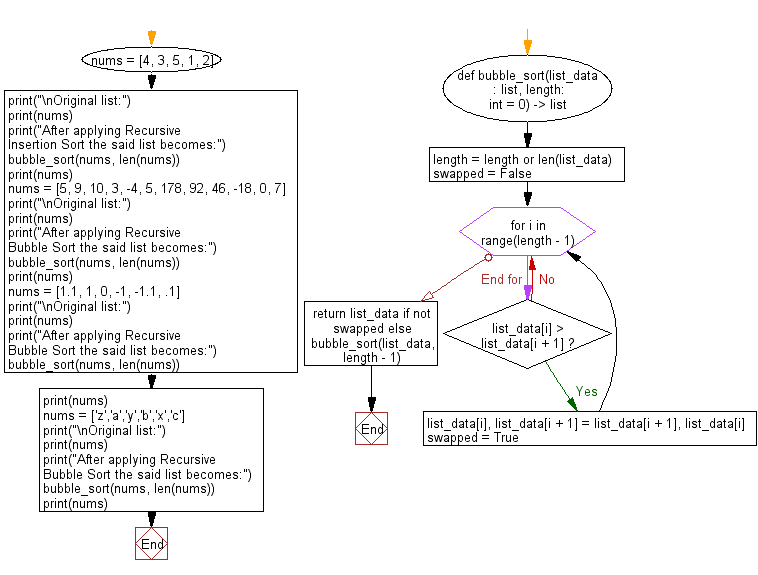﻿ Python: Sort unsorted numbers using Recursive Bubble Sort - w3resource# Python: Sort unsorted numbers using Recursive Bubble Sort

## Python Search and Sorting : Exercise-30 with Solution

Write a Python program to sort unsorted numbers using Recursive Bubble Sort.

Sample Solution:

Python Code:

``````#Ref.https://bit.ly/3oneU2l
def bubble_sort(list_data: list, length: int = 0) -> list:
length = length or len(list_data)
swapped = False
for i in range(length - 1):
if list_data[i] > list_data[i + 1]:
list_data[i], list_data[i + 1] = list_data[i + 1], list_data[i]
swapped = True

return list_data if not swapped else bubble_sort(list_data, length - 1)
nums = [4, 3, 5, 1, 2]
print("\nOriginal list:")
print(nums)
print("After applying Recursive Insertion Sort the said list becomes:")
bubble_sort(nums, len(nums))
print(nums)
nums = [5, 9, 10, 3, -4, 5, 178, 92, 46, -18, 0, 7]
print("\nOriginal list:")
print(nums)
print("After applying Recursive Bubble Sort the said list becomes:")
bubble_sort(nums, len(nums))
print(nums)
nums = [1.1, 1, 0, -1, -1.1, .1]
print("\nOriginal list:")
print(nums)
print("After applying Recursive Bubble Sort the said list becomes:")
bubble_sort(nums, len(nums))
print(nums)
nums = ['z','a','y','b','x','c']
print("\nOriginal list:")
print(nums)
print("After applying Recursive Bubble Sort the said list becomes:")
bubble_sort(nums, len(nums))
print(nums)
```
```

Sample Output:

```Original list:
[4, 3, 5, 1, 2]
After applying Recursive Insertion Sort the said list becomes:
[1, 2, 3, 4, 5]

Original list:
[5, 9, 10, 3, -4, 5, 178, 92, 46, -18, 0, 7]
After applying Recursive Bubble Sort the said list becomes:
[-18, -4, 0, 3, 5, 5, 7, 9, 10, 46, 92, 178]

Original list:
[1.1, 1, 0, -1, -1.1, 0.1]
After applying Recursive Bubble Sort the said list becomes:
[-1.1, -1, 0, 0.1, 1, 1.1]

Original list:
['z', 'a', 'y', 'b', 'x', 'c']
After applying Recursive Bubble Sort the said list becomes:
['a', 'b', 'c', 'x', 'y', 'z']
```

Flowchart:Python Code Editor:

What is the difficulty level of this exercise?

Test your Programming skills with w3resource's quiz.

﻿

## Python: Tips of the Day

Use Reversed() In for Loops:

```>>> tasks = ['laundry', 'picking up kids', 'gardening', 'cooking']